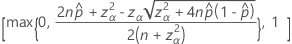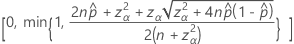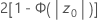# Methods and formulas for 1 Proportion

Select the method of your choice.

## Confidence interval (CI) for the exact method

The interval (PL, PU) is a 100(1 – α)% confidence interval of p. When x = 0 or x = n, Minitab calculates only a one-sided confidence interval.

### Lower limit

#### Notation

TermDescription
v1 2x
v2 2(nx + 1)
x number of events
n number of trials
F lower α/2 point of F distribution with v1 and v2 degrees of freedom

### Upper limit

#### Notation

TermDescription
v1 2(x + 1)
v2 2(nx)
x number of events
n number of trials
F upper α/2 point of F distribution with v1 and v2 degrees of freedom

## Confidence interval (CI) for the normal approximation

### Notation

TermDescriptionobserved probability,= x / n
x observed number of events in n trials
n number of trials
zα/2 inverse cumulative probability of the standard normal distribution at 1–α/2
α 1 – confidence level/100

## Exact test

### Formula

The sample (X) comes from binomial distribution with parameters n and p.
• H1: p > po, p-value = P{ X > x | p = po}
• H1: p < po, p-value = P{ X < x | p = po}
• H1: ppo and po = 1/2, p-value = P{ X < y or X > ny | p = po}

### Notation

TermDescription
n number of trials
p probability of success
x observed number of successes
y min {x, n x}

## Likelihood ratio test

### Formula

Minitab uses the likelihood ratio test for:

H1: p ≠ po and po ≠ 1/2

The likelihood function is defined as:

such that

LR (x) ≥ c

Minitab evaluates the likelihood ratio for all possible values of X = (0, 1,…, n) and sums the probabilities for all values for which the LR (y) ≥ LR (x).
• p-value = Σ P{X = y | p = po}

### Notation

TermDescription
c critical value chosen to obtain the desired significance level, α
x observed number of successes
y min {x, nx}
n number of trials

## Test statistic for the normal approximation

### Notation

TermDescriptionobserved probability, x/n
x observed number of events in n trials
n number of trials
p0 hypothesized probability
By using this site you agree to the use of cookies for analytics and personalized content.  Read our policy